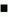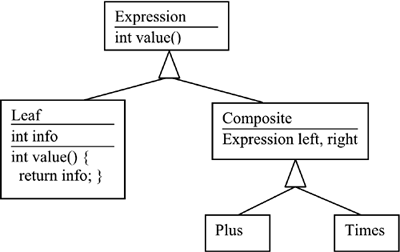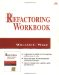# Challenges

 Exercise 13 Two Libraries. (Challenging). You're trying to integrate two modules from two different sources. Each module has its own logging approach. System A: ` package com.fubar.log; public final class Log { public int INFO=1, WARN=2, ERROR=3, FATAL=4; public static void setLog(File f) {...} public static void log(int level, String msg) {...} } ` Calls to Log.log are sprinkled throughout the code. System B: ` package com.bar.logger; public class Logger { public void informational(String msg) {...} public void informational(String msg, Exception e) {...} public void warning(String msg) {...} public void warning(String msg, Exception e) {...} public void fatal(String msg) {...} public void fatal(String msg, Exception e) {...} } public class LogFacility { public Logger makeLogger(String id) {...} public static void setOutput(OutputStream out) {...} } ` Objects that may need to log hold a Logger object. Your long- term goal is to move to the new standard logging facility in JDK 1.4, but your environment doesn't support that yet. What overall approach would you use to harmonize these classes with where you want to go? (Make sure to address the JDK 1.4 concern.) Create a simple test for each logger, and implement the logger with the simplest approach possible. Harmonize the classes so you can eliminate one of them. (Don't worry about the JDK 1.4 future yet.)See Appendix A for solutions. Exercise 14 Properties. (Online at www.xp123.com/rwb ) ` public void getTimes(Properties props) throws Exception { String valueString; int value; valueString = props.getProperty("interval"); if (valueString == null) { throw new MissingPropertiesException("monitor interval"); } value = Integer.parseInt(valueString); if (value <= 0) { throw new MissingPropertiesException("monitor interval > 0"); } checkInterval = value; valueString = props.getProperty("duration"); if (valueString == null) { throw new MissingPropertiesException("duration"); } value = Integer.parseInt(valueString); if (value <= 0) { throw new MissingPropertiesException("duration > 0"); } if ((value % checkInterval) != 0) { throw new MissingPropertiesException("duration % checkInterval"); } monitorTime = value; valueString = props.getProperty("departure"); if (valueString == null) { throw new MissingPropertiesException("departure offset"); } value = Integer.parseInt(valueString); if (value <= 0) { throw new MissingPropertiesException("departure > 0"); } if ((value % checkInterval) != 0) { throw new MissingPropertiesException("departure % checkInterval"); } departureOffset = value; } ` How would you handle the duplication? Notice that determination of the checkInterval doesn't involve % .See Appendix A for solutions. Exercise 15 Template Example. (originally in Extreme Programming Explored ). (Online at www.xp123.com/rwb ) ` try { String template = new String(sourceTemplate); // Substitute for %CODE% int templateSplitBegin = template.indexOf("%CODE%"); int templateSplitEnd = templateSplitBegin + 6; String templatePartOne = new String( template.substring(0, templateSplitBegin)); String templatePartTwo = new String( template.substring(templateSplitEnd, template.length())); code = new String(reqId); template = new String(templatePartOne + code + templatePartTwo); // Substitute for %ALTCODE% templateSplitBegin = template.indexOf("%ALTCODE%"); templateSplitEnd = templateSplitBegin + 9; templatePartOne = new String( template.substring(0, templateSplitBegin)); templatePartTwo = new String( template.substring(templateSplitEnd, template.length())); altcode = code.substring(0,5) + "-" + code.substring(5,8); out.print(templatePartOne + altcode + templatePartTwo); } catch (Exception e) { System.out.println("Error in substitute()"); } ` What duplication do you see? What would you do to remove the duplication? One piece that repeats is a structure of the form new String (some other string ). What does this code do? What does this have to do with the intern() method on class String? Does it apply here?See Appendix A for solutions. Exercise 16 Duplicate Observed Data. (Challenging). The refactoring Duplicate Observed Data works like this: If you have domain data in a widget-type class, move the domain data to a new domain class, and set up an observer so that the widget is notified of any changes to it. Thus, we've taken a situation where data was in one place, and we have not only duplicated it, but we've also added a need for synchronization between two objects. Why is this duplication considered acceptable (desirable, even)? (Hint: Your answer should touch on the Observer or Model-View-Controller pattern.) What are the performance implications of this approach?See Appendix A for solutions. Exercise 17 Java Libraries. The Java libraries have several places where there is duplication. Describe some examples of this. They might be at a low, medium, or high level. Why does this duplication exist? Is it worth it?See Appendix A for solutions. Exercise 18 Points. Suppose you see these two classes: ` public class Bird { // ... public void move(Point vector) { x += vector.x % maxX; y += vector.y % maxY; } } public class Button { // ... public void setPosition(Point p) { x = p.x; while (x >= maxX) x -= maxX; while (x < 0) x += maxX; y = p.y; while (y >= maxY) y -= maxY; while (y < 0) y += maxY; } } ` What is the duplication? What could you do to eliminate duplication in these two classes? Sometimes, two versions of duplicated code are similar, but one has fixed a bug and the other hasn't. How can refactoring help you in this situation?See Appendix A for solutions. Exercise 19 Expression. Suppose we have an expression that is structured in tree form. So the tree for 3 + 4 * 5 might look like this: ` + / \ 3 * / \ 4 5 ` The class hierarchy might look like this:The code for Plus.value() : ` public int value() { int v1 = left.value(); int v2 = right.value(); return v1 + v2; } ` The code for Times.value() : ` public int value() { int v1 = left.value(); int v2 = right.value(); return v1 * v2; } ` What steps would you take to reduce the duplication?See Appendix A for solutions.Refactoring Workbook
ISBN: 0321109295
EAN: 2147483647
Year: 2003
Pages: 146

Similar book on Amazon